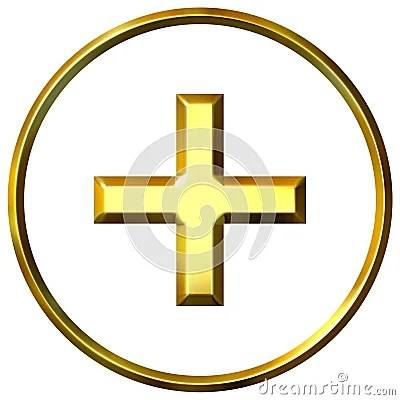## tarot correspondences

This is the list of number/tarot card correspondences:

1 = Magician
2 = High Priestess
3 = Empress
4 = Emperor
5 = Hierophant
6 = Lovers
7 = Chariot
8 = Strength
9 = Hermit
10 = Wheel of Fortune
11 = Justice
12 = Hanged Man
13 = Death
14 = Temperance
15 = Devil
16 = Tower
17 = Star
18 = Moon
19 = Sun
20= Judgement
21 = World
22 = Fool
23 = King of Wands
24 = Queen of Wands
25 = Knight of Wands
26 = Page of Wands
27 = Ace of Wands
28 = Two of Wands
29 = Three of Wands
30 = Four of Wands
31 = Five of Wands
32 = Six of Wands
33 = Seven of Wands
34 = Eight of Wands
35 = Nine of Wands
36 = Ten of Wands
37 = King of Cups
38 = Queen of Cups
39 = Knight of Cups
40 = Page of Cups
41 = Ace of Cups
42 = Two of Cups
43 = Three of Cups
44 = Four of Cups
45 = Five of Cups
46 = Six of Cups
47 = Seven of Cups
48 = Eight of Cups
49 = Nine of Cups
50 = Ten of Cups
51 = King of Swords
52 = Queen of Swords
53 = Knight of Swords
54 = Page of Swords
55 = Ace of Swords
56 = Two of Swords
57 = Three of Swords
58 = Four of Swords
59 = Five of Swords
60 = Six of Swords
61 = Seven of Swords
62 = Eight of Swords
63 = Nine of Swords
64 = Ten of Swords
65 = King of Pentacles
66 = Queen of Pentacles
67 = Knight of Pentacles
68 = Page of Pentacles
69 = Ace of Pentacles
70 = Two of Pentacles
71 = Three of Pentacles
72 = Four of Pentacles
73 = Five of Pentacles
74 = Six of Pentacles
75 = Seven of Pentacles
76 = Eight of Pentacles
77 = Nine of Pentacles
78 = Ten of Pentacles

79 = anger management80 = dealing with the aftermath

81 = revival

82 = jackpot

83 = a call for social justice

84 = leaving work85 = one step closer86 = high priced liasons87 = trappings of wealth88 = a real live wire

89 = visually inspiring

90 = it ends where it all began91 = timing

92 = legal dealings93 = making the best of a worst-case scenario94 = airing grievances95 = getting others to overcome their self-interest96 = ethical money making97 = humility98 = audibly inspiring

99 = way out there

—————————————————————————————-100 = an army of one

### One Response to “tarot correspondences”

1. […] The Tarot numerology correspondence chart by Ed Peterson: https://numerologybasics.com/tarot-correspondences/ […]